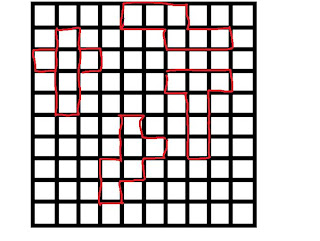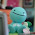## Tuesday, March 15, 2011

### Paulo's Surface Area of a Prism

Homewor
pg 54, question 2

2. Calculate the surface area of each rectangular prism to the nearest tenth of a centimetre squared.Front:
5x11.5=57.5cm2
5x11.5=57.5cm2

Top:
11.5x3.2=36.8cm2
11.5x3.2=36.8cm2

Side:
5x3.2=16cm2
5x3.2=16cm2
---------------
TSA 220.6cm2

b)
Front:
12x10.4=124.8cm2
12x10.4=124.8cm2

Top:
10.4x4.5=46.8cm2
10.4x4.5=46.8cm2

Side:
5x3.2=16cm2
5x3.2=16cm2
----------------
TSA 375.2 cm2

pg 54, question 3

3

a)
triangle+ 7x8/2= 28cm2
triangle+ 7x8/2=28cm2

a=2.5x8=20cm2
a=2.5x7=17.5cm2
a=2.5x=10.6=26.5cm2
------------------------
TSA 120cm2

pg 53, question 43.14x4.1x4.1)x11

#### 1 comment:

1.Good Job Paulo. I like your post, it made sense. You should add some colour to highlight the important parts or unless it gets boring to the eye. Another thing is to make your picture easier, because I found that really dufficult. Other than that, great job.